# Ring of polynomials

(diff) ← Older revision | Latest revision (diff) | Newer revision → (diff)
Jump to: navigation, search

polynomial ring

A ring whose elements are polynomials (cf. Polynomial) with coefficients in some fixed field. Rings of polynomials over an arbitrary commutative associative ring, for example, over the ring of integers, are also discussed. The accepted notation for the ring of polynomials in a finite set of variablesoveris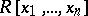. It is possible to speak of a ring of polynomials in an infinite set of variables if it is assumed that each individual polynomial depends only on a finite number of variables. A ring of polynomials over a ringis a (commutative) free algebra with an identity over; the set of variables serves as a system of free generators of this algebra.

A ring of polynomials over an arbitrary integral domain is itself an integral domain. A ring of polynomials over a factorial ring is itself factorial.

For a ring of polynomials in a finite number of variables over a fieldthere is Hilbert's basis theorem: Every ideal inis finitely generated (as an ideal) (cf. Hilbert theorem). A ring of polynomials in one variable over a field,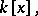is a principal ideal ring, that is, each ideal of it is generated by one element. Moreover,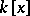is a Euclidean ring. This property ofgives one the possibility of comprehensively describing the finitely-generated modules over it and, in particular, of reducing linear operators in a finite-dimensional vector space to canonical form (see Jordan matrix). Forthe ring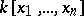is not a principal ideal ring.

Letbe a commutative associative-algebra with an identity, and letbe an element of the Cartesian power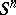. Then there is a unique-algebra homomorphism of the ring of polynomials invariables into,for which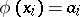, for all, andis the identity of. The image of a polynomialunder this homomorphism is called its value at the point. A point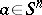is called a zero of a system of polynomials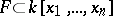if the value of each polynomial fromat this point is. For a ring of polynomials there is Hilbert's Nullstellen Satz: Letbe an ideal in the ring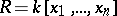, letbe the set of zeros ofin, where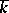is the algebraic closure of, and letbe a polynomial invanishing at all points of. Then there is a natural numbersuch that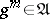(cf. Hilbert theorem).

Letbe an arbitrary module over the ring. Then there are free-modules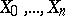and homomorphisms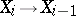such that the sequence of homomorphisms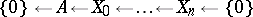is exact, that is, the kernel of one homomorphism is the image of the next. This result is one possible formulation of the Hilbert theorem on syzygies for a ring of polynomials.

A finitely-generated projective module over a ring of polynomials in a finite number of variables with coefficients from a principal ideal ring is free (see , ); this is the solution of Serre's problem.

Only in certain particular cases are there answers to the following questions: 1) Is the group of automorphisms of a ring of polynomials generated by elementary automorphisms? 2) Isgenerated by some setfor which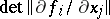is a non-zero constant? 3) Ifis isomorphic to, mustbe isomorphic to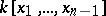?

How to Cite This Entry:
Ring of polynomials. Encyclopedia of Mathematics. URL: http://encyclopediaofmath.org/index.php?title=Ring_of_polynomials&oldid=14262
This article was adapted from an original article by Yu.A. Bakhturin (originator), which appeared in Encyclopedia of Mathematics - ISBN 1402006098. See original article